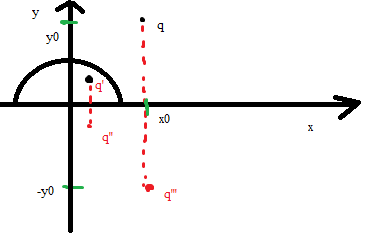# Problem based on conducting planeMaybe it can it seems to me that this task is solved quite easily by using images method?

3 лайка

Yeah, you just need to make sure that the set of image charges produces an equipotential surface of the plate

2 лайка

oh so what answer are you getting for potential?

The answer is a little scary, but In general, it looks like this:

\phi=\frac{kq}{\sqrt{(x-x_0)^2+(y+y_0)^2+z^2}}-\frac{kq}{\sqrt{(x-x_0)^2+(y-y_0)^2+z^2}}-\frac{kqa}{b\sqrt{(x-\displaystyle\frac{a^2 x_0}{b^2})^2+(y+\displaystyle\frac{a^2 x_0}{b^2})^2+z^2}}+\frac{kqa}{b\sqrt{(x-\displaystyle\frac{a^2 x_0}{b^2})^2+(y-\displaystyle\frac{a^2 x_0}{b^2})^2+z^2}}+
1 лайк

ah thank you

@Alisher @Miras what answer are you getting in electrostatics force?